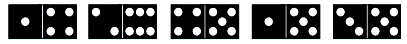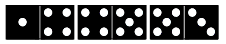#CodeStepByStep## chainExists

Language/Type: C++ recursion backtracking

Write a recursive function named `chainExists` that looks for domino number chains. The game of Dominoes is played with rectangular pieces composed of two connected squares, each of which is marked with a certain number of dots. For example, each of the following five rectangles represents a domino:Dominoes are connected end-to-end to form chains, subject to the condition that two dominoes can be linked together only if the numbers match, although it is legal to rotate dominoes 180 degrees so that the numbers are reversed. For example, you could connect the first, third, and fifth dominoes in the above collection to form the following chain. Note that the 3-5 domino had to be rotated so that it matched up correctly with the 4-5.Given a set of dominoes, an interesting question to ask is whether it is possible to form a chain starting at one number and ending with another. For example, the example chain shown earlier makes it clear that you can use the original set of five dominoes to build a chain starting with a 1 and ending with a 3. Similarly, if you wanted to build a chain starting with a 6 and ending with a 2, you could do so using only one domino. On the other hand, there is no way, using just these five dominoes, to build a chain starting with a 1 and ending with a 6.

Dominoes can be represented in C++ as a structure with a pair of integers. Assuming the type `Domino` is defined as:

```struct Domino {
int first;
int second;
};
```

For this problem, write a function named `chainExists` that accepts three parameters: a reference to a `vector` of `Domino` objects, and a starting and ending integer value. Your function should return `true` if it is possible to build a chain from start to finish using any subset of the dominoes in the dominoes vector. To simplify the problem, assume that `chainExists` always returns `true` if the start integer is equal to the finish integer, because you can trivially connect any number to itself with a chain of zero dominoes. (Your function doesn't need to report the chain's elements; worry only about the yes or no that comes back in the form of a `bool`.) For example, if passed the domino set illustrated above, `chainExists` should produce the following results:

Call Value Returned
`chainExists(dominoes, 1, 3)` `true`
`chainExists(dominoes, 5, 5)` `true`
`chainExists(dominoes, 1, 6)` `false`

If the value of the start or end integer passed is negative, your function should throw an integer exception.

Constraints: You are allowed to modify the vector passed in as the parameter as you compute the answer, as long as you restore it to its original form by the time the overall call is finished. You may use loops in solving this problem if you like, but your overall solution must use recursive backtracking. You may define helper functions if you like.

Function: Write a C++ function as described, not a complete program.